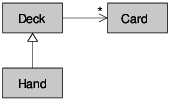# 9.8: Class Diagrams

$$\newcommand{\vecs}{\overset { \rightharpoonup} {\mathbf{#1}} }$$ $$\newcommand{\vecd}{\overset{-\!-\!\rightharpoonup}{\vphantom{a}\smash {#1}}}$$$$\newcommand{\id}{\mathrm{id}}$$ $$\newcommand{\Span}{\mathrm{span}}$$ $$\newcommand{\kernel}{\mathrm{null}\,}$$ $$\newcommand{\range}{\mathrm{range}\,}$$ $$\newcommand{\RealPart}{\mathrm{Re}}$$ $$\newcommand{\ImaginaryPart}{\mathrm{Im}}$$ $$\newcommand{\Argument}{\mathrm{Arg}}$$ $$\newcommand{\norm}{\| #1 \|}$$ $$\newcommand{\inner}{\langle #1, #2 \rangle}$$ $$\newcommand{\Span}{\mathrm{span}}$$ $$\newcommand{\id}{\mathrm{id}}$$ $$\newcommand{\Span}{\mathrm{span}}$$ $$\newcommand{\kernel}{\mathrm{null}\,}$$ $$\newcommand{\range}{\mathrm{range}\,}$$ $$\newcommand{\RealPart}{\mathrm{Re}}$$ $$\newcommand{\ImaginaryPart}{\mathrm{Im}}$$ $$\newcommand{\Argument}{\mathrm{Arg}}$$ $$\newcommand{\norm}{\| #1 \|}$$ $$\newcommand{\inner}{\langle #1, #2 \rangle}$$ $$\newcommand{\Span}{\mathrm{span}}$$$$\newcommand{\AA}{\unicode[.8,0]{x212B}}$$

So far we have seen stack diagrams, which show the state of a program, and object diagrams, which show the attributes of an object and their values. These diagrams represent a snapshot in the execution of a program, so they change as the program runs.

They are also highly detailed; for some purposes, too detailed. A class diagram is a more abstract representation of the structure of a program. Instead of showing individual objects, it shows classes and the relationships between them.

There are several kinds of relationship between classes:

• Objects in one class might contain references to objects in another class. For example, each Rectangle contains a reference to a Point, and each Deck contains references to many Cards. This kind of relationship is called HAS-A, as in, “a Rectangle has a Point.”
• One class might inherit from another. This relationship is called IS-A, as in, “a Hand is a kind of a Deck.”
• One class might depend on another in the sense that changes in one class would require changes in the other.

A class diagram is a graphical representation of these relationships. For example, Figure 18.8.1 shows the relationships between Card, Deck and Hand.Figure $$\PageIndex{1}$$: Class diagram.

The arrow with a hollow triangle head represents an IS-A relationship; in this case it indicates that Hand inherits from Deck.

The standard arrow head represents a HAS-A relationship; in this case a Deck has references to Card objects.

The star (*) near the arrow head is a multiplicity; it indicates how many Cards a Deck has. A multiplicity can be a simple number, like 52, a range, like 5..7 or a star, which indicates that a Deck can have any number of Cards.

A more detailed diagram might show that a Deck actually contains a list of Cards, but built-in types like list and dict are usually not included in class diagrams.

Exercise $$\PageIndex{1}$$

Read TurtleWorld.py, World.py and Gui.py and draw a class diagram that shows the relationships among the classes defined there.

This page titled 9.8: Class Diagrams is shared under a CC BY-NC-SA 3.0 license and was authored, remixed, and/or curated by Allen B. Downey (Green Tea Press) .